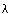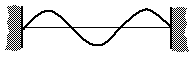TUTORIAL QUESTIONS 115-125

115.  For a standing wave on a string,
a) you can have anyand any f so long as the relationship, v = fholds.
b) only certain wavelengths and frequencies are allowed
c) the wave velocity is totally determined by the medium
d) the wave velocity depends on the dynamic and inertial parameters
e) a and c only
f) b, c and d only
g) a, c and d only
h) none of the above

Fundamentals of Sound reference: Sec. 2-C.

120.  Consider the diagram below for a standing wave on a string 9 ft long that is tied at both ends.  The wave velocity in the string is 16 ft/s.What is the wavelength?

Fundamentals of Sound reference: Sec. 2-C.

125.  Consider again the diagram in Question 120 for a standing wave on a string 9 ft long that is tied at both ends.

Which mode is this?

a) 2nd Harmonic (and no other answer is correct)
b) 3rd Harmonic ( and no other answer is correct)
c) 2nd Overtone( and no other answer is correct)
d) 3rd Overtone (and no other answer is correct)
e) Both a and c
f) Both b and d
g) Both b and c

Fundamentals of Sound reference: Sec. 2-C.

Back to Tutorial Listing